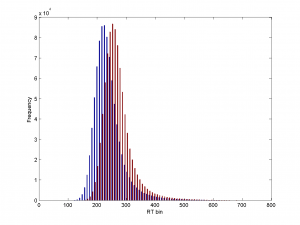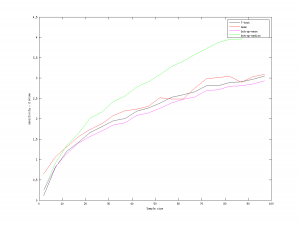Categories

# Bootstrap update

Update: This post used an incorrect implementation of the bootstrap, so the conclusions don’t hold. See this correction

Mike suggested that I alter the variance of the underlying distibutions. This makes total sense, since it matches what we are usually trying to do in psychological research – detect a small difference in a lot of noise. So I made the underlying distibutions look a lot like reaction time distributions, with a 30ms difference between them. The code is

```    t0=200;
s1=t0+25*(randn(1,m)+exp(randn(1,m)));
s2=t0+25*(randn(1,m)+exp(randn(1,m)))+d;
```

Where m is the sample size, and d is either 0 or 30. For a very large sample, the distributions look like this:After a discussion with Jim I looked at the hit rate and false alarm rate separately. For the simple comparison of means, the false alarm rate stays around 0.5 (as you’d predict). For the other tests it drops to about 0.05. The simple comparison of means is so sensitive to a true difference, however, that the dprime can still be superior to that of the other tests. Which suggests dprime is not a good summary statistic to me, rather than that we should do testing simply by comparing the sample means.

So I rerun the procedure I described before, but with higher variance on the underlying samples.The results are very similar. The bootstrap using the mean as the test statistic is worse than the t-test. The bootstrap using the median is clear superior. This surprises me. I had been told that the bootstrap was superior for nonparametric distributions. In this case it seems as if using the mean as a test statistic eliminates the potential superiority of bootstrapping.

This is still a work in progress, so I will investigate further and may have to update this conclusion as the story evolves.

## 4 replies on “Bootstrap update”Andysays:

What’s a nonparametric distribution?

In this context, it is a non-normal distribution, which is what I should have said of courseAndy Fugardsays:

[…] previously on this blog (here, and ) I was playing around with the bootstrap as a way of testing if two samples are drawn from a […]